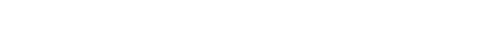glAccum.3glOpenGL man pages

```

```

## Name

```  glAccum - operate on the accumulation	buffer

```

## C Specification

```  void glAccum(	GLenum op,
GLfloat	value )

```

## Parameters

```
op	 Specifies the accumulation buffer operation.  Symbolic	constants

value	 Specifies a floating-point value used in the accumulation buffer
operation.  op	determines how value is	used.

```

## Description

```  The accumulation buffer is an	extended-range color buffer.  Images are not
rendered into	it.  Rather, images rendered into one of the color buffers
are added to the contents of the accumulation	buffer after rendering.
Effects such as antialiasing (of points, lines, and polygons), motion	blur,
and depth of field can be created by accumulating images generated with
different transformation matrices.

Each pixel in	the accumulation buffer	consists of red, green,	blue, and
alpha	values.	 The number of bits per	component in the accumulation buffer
depends on the implementation. You can examine this number by	calling
glGetIntegerv	four times, with arguments GL_ACCUM_RED_BITS,
GL_ACCUM_GREEN_BITS, GL_ACCUM_BLUE_BITS, and GL_ACCUM_ALPHA_BITS,
respectively.	 Regardless of the number of bits per component, however, the
range	of values stored by each component is [-1, 1].	The accumulation
buffer pixels	are mapped one-to-one with frame buffer	pixels.

glAccum operates on the accumulation buffer.	The first argument, op,	is a
symbolic constant that selects an accumulation buffer	operation.  The
second argument, value, is a floating-point value to be used in that
GL_MULT, and GL_RETURN.

All accumulation buffer operations are limited to the	area of	the current
scissor box and are applied identically to the red, green, blue, and alpha
components of	each pixel.  The contents of an	accumulation buffer pixel
component are	undefined if the glAccum operation results in a	value outside
the range [-1,1].  The operations are	as follows:

GL_ACCUM  Obtains R, G, B, and A values from the buffer currently selected
by 2n-1, where n is	the number of bits allocated to	each color
component in the currently selected	buffer.	 The result is a
floating-point value in the	range [0,1], which is multiplied by
value and added to the corresponding pixel component in the
accumulation buffer, thereby updating the accumulation buffer.

GL_LOAD   Similar to GL_ACCUM, except	that the current value in the
accumulation buffer	is not used in the calculation of the new
value.  That is, the R, G, B, and A	values from the	currently
selected buffer are	divided	by 2n-1, multiplied by value, and
then stored	in the corresponding accumulation buffer cell,
overwriting	the current value.

GL_ADD    Adds value to each R, G, B,	and A in the accumulation buffer.

GL_MULT   Multiplies each R, G, B, and A in the accumulation buffer by
value and returns the scaled component to its corresponding
accumulation buffer	location.

GL_RETURN Transfers accumulation buffer values to the	color buffer or
buffers currently selected for writing.  Each R, G,	B, and A
component is multiplied by value, then multiplied by 2n-1,
clamped to the range [0,2n-1], and stored in the corresponding
display buffer cell.  The only fragment operations that are
applied to this transfer are pixel ownership, scissor, dithering,

The accumulation buffer is cleared by	specifying R, G, B, and	A values to
set it to with the glClearAccum directive, and issuing a glClear command
with the accumulation	buffer enabled.

```

## Notes

```  Only those pixels within the current scissor box are updated by any glAccum
operation.

```

## Errors

```  GL_INVALID_ENUM is generated if op is	not an accepted	value.

GL_INVALID_OPERATION is generated if there is	no accumulation	buffer.

GL_INVALID_OPERATION is generated if glAccum is executed between the
execution of	glBegin	and the	corresponding execution	of glEnd.

```

## Associated Gets

```  glGet	with argument GL_ACCUM_RED_BITS
glGet	with argument GL_ACCUM_GREEN_BITS
glGet	with argument GL_ACCUM_BLUE_BITS
glGet	with argument GL_ACCUM_ALPHA_BITS

```

```  glBlendFunc, glClear,	glClearAccum, glCopyPixels, glGet, glLogicOp,